# GSEB Solutions Class 9 Maths Chapter 1 Number Systems Ex 1.3

Gujarat Board GSEB Textbook Solutions Class 9 Maths Chapter 1 Number Systems Ex 1.3 Textbook Questions and Answers.

## Gujarat Board Textbook Solutions Class 9 Maths Chapter 1 Number Systems Ex 1.3Question 1.
Write the following in decimal form and say what kind of decimal expansion each has
Solution:
(i) $$\frac { 36 }{ 100 }$$
(ii) $$\frac { 1 }{ 11 }$$
(iii) 4$$\frac { 1 }{ 8 }$$
(iv) $$\frac { 3 }{ 13 }$$
(v) $$\frac { 2 }{ 11 }$$
(vi) $$\frac { 329 }{ 400 }$$
Solution:
(i) We have
$$\frac { 36 }{ 100 }$$ = 0.36
∴ The decimal expansion is terminating.

(ii) We have $$\frac { 1 }{ 11 }$$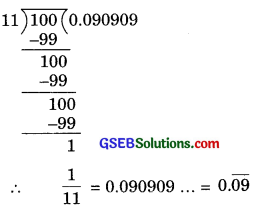The decimal expansion is non-terminating repeating.

(iii) We have 4$$\frac { 1 }{ 8 }$$ = $$\frac { 33 }{ 8 }$$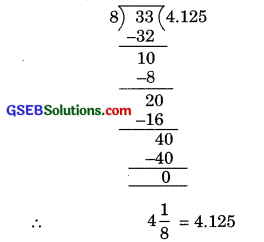The decimal expansion is terminating.

(iv) We have $$\frac { 3 }{ 13 }$$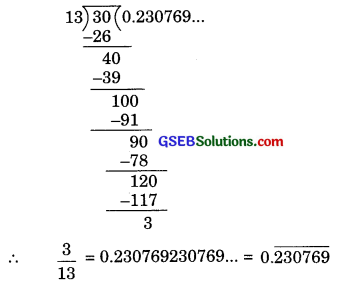The decimal expansion is non-terminating repeating.

(v) We have $$\frac { 2 }{ 11 }$$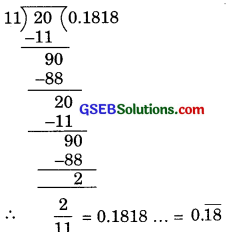The decimal expansion is non-terminating repeating.

(vi) We have $$\frac { 329 }{ 400 }$$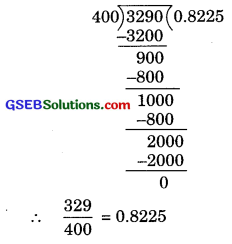The decimal expansion is terminating.

Question 2.
You know that $$\frac { 1 }{ 7 }$$ = 0.$$\overline { 142857 }$$. Can you predict what the decimal expansions of $$\frac { 2 }{ 7 }$$, $$\frac { 3 }{ 7 }$$, $$\frac { 4 }{ 7 }$$, $$\frac { 5 }{ 7 }$$, $$\frac { 6 }{ 7 }$$ are, without actually doing the long division? If so, how?
(Hint. Study the remainders while finding the value of $$\frac { 1 }{ 7 }$$ carefully.)
Solution:
Yes, we can predict the decimal expansions of $$\frac { 2 }{ 7 }$$, $$\frac { 3 }{ 7 }$$, $$\frac { 4 }{ 7 }$$, $$\frac { 5 }{ 7 }$$, $$\frac { 6 }{ 7 }$$ without actually doing the long division.
We know $$\frac { 1 }{ 7 }$$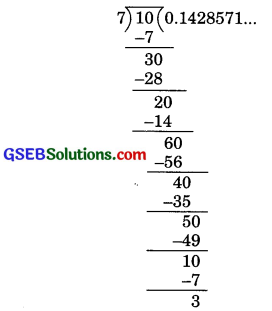$$\frac { 1 }{ 7 }$$ = 0.142857142857…
∴ $$\frac { 1 }{ 7 }$$ = 0.$$\overline { 142857 }$$
Now
$$\frac { 2 }{ 7 }$$ = 2 x $$\frac { 1 }{ 7 }$$ = 2 x 0.$$\overline { 142857 }$$
∴ $$\frac { 2 }{ 7 }$$ = 0.$$\overline { 285714 }$$
Similarly,$$\frac { 3 }{ 7 }$$ = 3 x $$\frac { 1 }{ 7 }$$ = 3 x 0.$$\overline { 142857 }$$
⇒ $$\frac { 3 }{ 7 }$$ = 0.$$\overline { 428571 }$$
$$\frac { 4 }{ 7 }$$ = 4 x $$\frac { 1 }{ 7 }$$ = 4 x 0.$$\overline { 142857 }$$
⇒ $$\frac { 4 }{ 7 }$$ = 0.$$\overline { 571428 }$$
$$\frac { 5 }{ 7 }$$ = 5 x $$\frac { 1 }{ 7 }$$ = 5 x 0.$$\overline { 142857 }$$
⇒ $$\frac { 5}{ 7 }$$ = 0.$$\overline { 714285 }$$
$$\frac { 6 }{ 7 }$$ = 6 x $$\frac { 1 }{ 7 }$$ = 6 x 0.$$\overline { 142857 }$$
⇒ $$\frac { 6}{ 7 }$$ = 0.$$\overline { 857142 }$$Question 3.
Express the following in the form $$\frac { p }{ q }$$, where p and q are integers and q ≠ 0.
(i) 0.$$\overline { 6 }$$
(ii) 0.4$$\overline { 7 }$$
(iii) 0.$$\overline { 0.001 }$$
Solution:
(i) 0.$$\overline { 6 }$$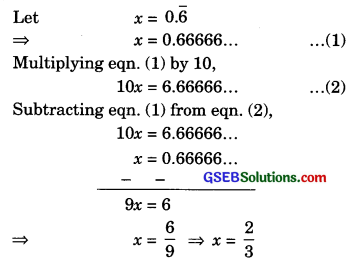(ii) We have 0.4$$\overline { 7 }$$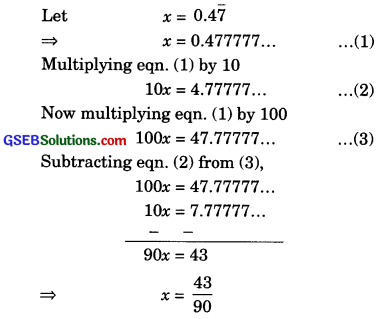(iii) We have 0.$$\overline { 0.001 }$$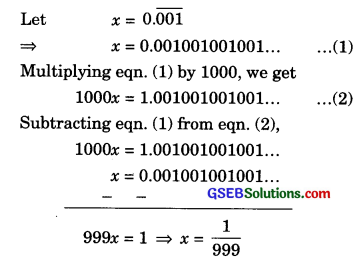Question 4.
Express 0.99999… in the form of $$\frac { p }{ q }$$. Are you surprised by your answer? With your teacher and classmates discuss why the answer makes sense.
Solution:
Let x = 0.99999… …(1)
Multiplying by 10 on both sides, we get
10x = 9.99999… …(2)
Subtracting equation (1) from eqn. (2),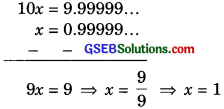Thus 0.99999… = 1 = $$\frac { 1 }{ 1 }$$
Here we get p = 1 and q = 1
Since 0.99999… goes on forever. Hence there is no gap between 1 and 0.99999… and hence both are equal.

Question 5.
What can the maximum number of digits be in the repeating block of digits in the decimal expansion of $$\frac { 1 }{ 17 }$$? Perform the division to check your answer.
Solution:
Long division method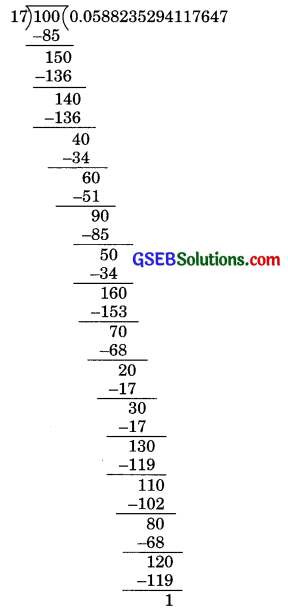Thus $$\frac { 1 }{ 17 }$$ = 0.$$\overline { 0.0588235294117647 }$$
We observe that by long division method maximum number of digits in repeating block in the decimal expansion of $$\frac { 1 }{ 17 }$$ is 16, thus answer is verified.

Question 6.
Look at several examples of rational numbers in the form $$\frac { p }{ q }$$ (q ≠ 0) where p and q are integers with no common factors other than 1 and having terminating decimal representations (expansions). Can you guess what property q must satisfy?
Solution:
(i) $$\frac { 1 }{ 2 }$$ = $$\frac { 1 × 5 }{ 2 × 5 }$$ = $$\frac { 5 }{ 10 }$$ = 0.5

(ii) $$\frac { 3 }{ 4 }$$ = $$\frac { 3×5 × 5 }{ 2 × 2 × 5 × 5 }$$ = $$\frac { 75 }{ 100 }$$ = 0.75

(iii) $$\frac { 7 }{ 8 }$$ = $$\frac { 7 × 5 × 5 × 5 }{ 2 × 2 × 2 × 5 × 5 × 5 }$$ = $$\frac { 875 }{ 1000 }$$ = 0.875

(iv) $$\frac { 13 }{ 25 }$$ = $$\frac { 13 × 2 × 2 }{ 5 × 5 × 2 × 2 }$$ = $$\frac{52}{5^{2} \times 2^{2}}$$
= $$\frac{52}{(10)^{2}}$$ = $$\frac { 52 }{ 100 }$$ = 0.52

(v) $$\frac { 3 }{ 125 }$$ = $$\frac { 3 }{ 5 × 5 × 5 }$$ = = $$\frac{3}{5^{3}}$$ = $$\frac{3 \times 2^{3}}{5^{3} \times 2^{3}}$$
= $$\frac{3 \times 8}{(5 \times 2)^{3}}$$ = $$\frac { 24 }{ 1000 }$$ = 0.024

(vi) $$\frac { 27 }{ 16 }$$ = $$\frac{27 \times 5^{4}}{2^{4} \times 5^{4}}$$ = $$\frac{27 \times 5^{4}}{(2 \times 5)^{4}}$$
= $$\frac{27×625}{(10)^{4}}$$ = $$\frac{16875}{(10)^{4}}$$ = 1.6875
We observe that the denominator of all the above rational numbers are of the form 2m x 5n i.e., the prime factorization of denominators has only powers of 2 or powers of 5 or both.Question 7.
Write three numbers whose decimal expansions are non-terminating non-recurring.
Solution:
(i) 0.012012001200012…
(ii) 0.21021002100021000021…
(iii) 0.32032003200032000032…

Question 8.
Find three different irrational numbers between the rational numbers $$\frac { 5 }{ 7 }$$ and $$\frac { 9 }{ 11 }$$.
Solution:
$$\frac { 5 }{ 7 }$$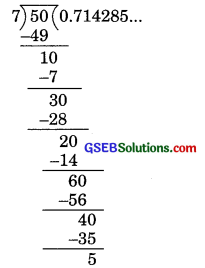Thus $$\frac { 5 }{ 7 }$$ = 0.714285…
$$\frac { 5 }{ 7 }$$ = 0.$$\overline { 714285 }$$….
Now $$\frac { 9 }{ 11 }$$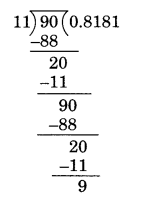Thus $$\frac { 9 }{ 11 }$$ = 0.8181… = 0.$$\overline { 81 }$$
Thus three irrational numbers between the rational numbers $$\frac { 5 }{ 7 }$$ and $$\frac { 9 }{ 11 }$$ can be taken as
0. 73073007300073000073…
0. 757075700757000757…
and 0.808008000800008…Question 9.
Classify the following numbers as rational or irrational.
(i) $$\sqrt{23}$$
(ii) $$\sqrt{225}$$
(iii) 0.3796
(iv) 7.478478…
(v) 1.101001000100001…
Solution:
(i) $$\sqrt{23}$$, 23 is not a perfect square so $$\sqrt{23}$$ will not give an integral value.
Hence it is not a rational number.

(ii) $$\sqrt{225}$$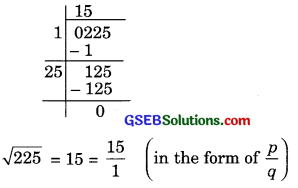∴ $$\sqrt{225}$$
Here p = 15
and q = 1 (q ≠ 0)

(iii) 0.3796
The decimal expression is terminating.
Hence 0.3796 is a rational number.

(iv) 7.478478…
∴ 7.478478… = 7.$$\overline { 748 }$$
The decimal expansion is non-terminating recurring.
∴ 7.478478… is a rational number.

(v) 1.101001000100001…
∵ The decimal expansion is non – terminating non-recurring.
∴ 1.101001000100001… is an irrational number.